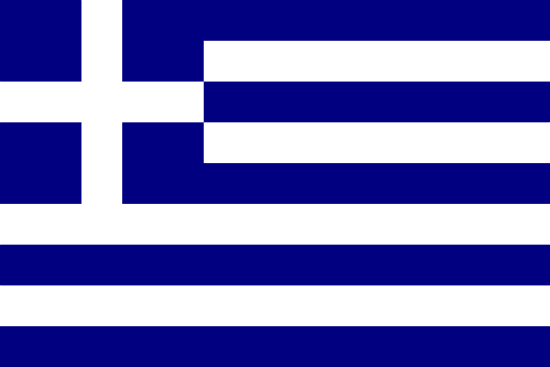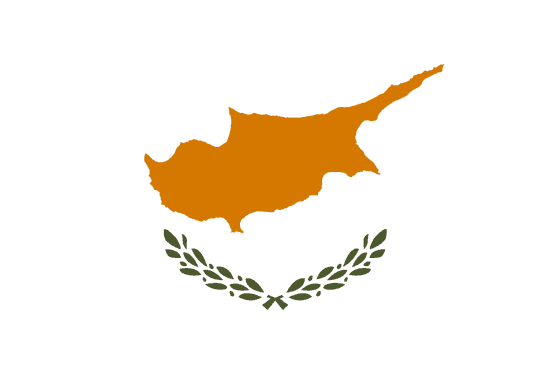字母（大、小写） 名称 现代发音（国际音标） Α α άλφα álpha [a] Β β βήτα bḗta [v] Γ γ γάμμα gámma /i/,/e/前为[ʝ]，其余为[ɣ] Δ δ δέλτα délta [ð] Ε ε έψιλον épsilon [e] Ζ ζ ζήτα zḗta [z] Η η ήτα ḗta [i] Θ θ θήτα thḗta [θ] Ι ι ιώτα iṓta [i] Κ κ κάππα káppa /i/,/e/前为[c]，其余为[k] Λ λ λάμδα lámda [l] Μ μ μυ my [m] Ν ν νυ ny [n] Ξ ξ ξι xi [ks] Ο ο όμικρον ómikron [o] Π π πι pi [p] Ρ ρ ρω rō [ɾ] Σ σ为词中／ς为词尾 σίγμα sígma [s] Τ τ ταυ tau [t] Υ υ ύψιλον ýpsilon [i] Φ φ φι phi [f] Χ χ χι chi /i/,/e/前为[ç]，其余为[x] Ψ ψ ψι psi [ps] Ω ω ωμέγα ōméga [o]